# sklearn.kernel_approximation.Nystroem¶

class sklearn.kernel_approximation.Nystroem(kernel='rbf', *, gamma=None, coef0=None, degree=None, kernel_params=None, n_components=100, random_state=None, n_jobs=None)[source]

Approximate a kernel map using a subset of the training data.

Constructs an approximate feature map for an arbitrary kernel using a subset of the data as basis.

Read more in the User Guide.

New in version 0.13.

Parameters:
kernelstr or callable, default=’rbf’

Kernel map to be approximated. A callable should accept two arguments and the keyword arguments passed to this object as kernel_params, and should return a floating point number.

gammafloat, default=None

Gamma parameter for the RBF, laplacian, polynomial, exponential chi2 and sigmoid kernels. Interpretation of the default value is left to the kernel; see the documentation for sklearn.metrics.pairwise. Ignored by other kernels.

coef0float, default=None

Zero coefficient for polynomial and sigmoid kernels. Ignored by other kernels.

degreefloat, default=None

Degree of the polynomial kernel. Ignored by other kernels.

kernel_paramsdict, default=None

Additional parameters (keyword arguments) for kernel function passed as callable object.

n_componentsint, default=100

Number of features to construct. How many data points will be used to construct the mapping.

random_stateint, RandomState instance or None, default=None

Pseudo-random number generator to control the uniform sampling without replacement of n_components of the training data to construct the basis kernel. Pass an int for reproducible output across multiple function calls. See Glossary.

n_jobsint, default=None

The number of jobs to use for the computation. This works by breaking down the kernel matrix into n_jobs even slices and computing them in parallel.

None means 1 unless in a joblib.parallel_backend context. -1 means using all processors. See Glossary for more details.

New in version 0.24.

Attributes:
components_ndarray of shape (n_components, n_features)

Subset of training points used to construct the feature map.

component_indices_ndarray of shape (n_components)

Indices of components_ in the training set.

normalization_ndarray of shape (n_components, n_components)

Normalization matrix needed for embedding. Square root of the kernel matrix on components_.

n_features_in_int

Number of features seen during fit.

New in version 0.24.

feature_names_in_ndarray of shape (n_features_in_,)

Names of features seen during fit. Defined only when X has feature names that are all strings.

New in version 1.0.

AdditiveChi2Sampler

Approximate feature map for additive chi2 kernel.

PolynomialCountSketch

Polynomial kernel approximation via Tensor Sketch.

RBFSampler

Approximate a RBF kernel feature map using random Fourier features.

SkewedChi2Sampler

Approximate feature map for “skewed chi-squared” kernel.

sklearn.metrics.pairwise.kernel_metrics

List of built-in kernels.

References

• Williams, C.K.I. and Seeger, M. “Using the Nystroem method to speed up kernel machines”, Advances in neural information processing systems 2001

• T. Yang, Y. Li, M. Mahdavi, R. Jin and Z. Zhou “Nystroem Method vs Random Fourier Features: A Theoretical and Empirical Comparison”, Advances in Neural Information Processing Systems 2012

Examples

>>> from sklearn import datasets, svm
>>> from sklearn.kernel_approximation import Nystroem
>>> X, y = datasets.load_digits(n_class=9, return_X_y=True)
>>> data = X / 16.
>>> clf = svm.LinearSVC()
>>> feature_map_nystroem = Nystroem(gamma=.2,
...                                 random_state=1,
...                                 n_components=300)
>>> data_transformed = feature_map_nystroem.fit_transform(data)
>>> clf.fit(data_transformed, y)
LinearSVC()
>>> clf.score(data_transformed, y)
0.9987...


Methods

 fit(X[, y]) Fit estimator to data. fit_transform(X[, y]) Fit to data, then transform it. get_feature_names_out([input_features]) Get output feature names for transformation. get_params([deep]) Get parameters for this estimator. set_output(*[, transform]) Set output container. set_params(**params) Set the parameters of this estimator. Apply feature map to X.
fit(X, y=None)[source]

Fit estimator to data.

Samples a subset of training points, computes kernel on these and computes normalization matrix.

Parameters:
Xarray-like, shape (n_samples, n_features)

Training data, where n_samples is the number of samples and n_features is the number of features.

yarray-like, shape (n_samples,) or (n_samples, n_outputs), default=None

Target values (None for unsupervised transformations).

Returns:
selfobject

Returns the instance itself.

fit_transform(X, y=None, **fit_params)[source]

Fit to data, then transform it.

Fits transformer to X and y with optional parameters fit_params and returns a transformed version of X.

Parameters:
Xarray-like of shape (n_samples, n_features)

Input samples.

yarray-like of shape (n_samples,) or (n_samples, n_outputs), default=None

Target values (None for unsupervised transformations).

**fit_paramsdict

Returns:
X_newndarray array of shape (n_samples, n_features_new)

Transformed array.

get_feature_names_out(input_features=None)[source]

Get output feature names for transformation.

The feature names out will prefixed by the lowercased class name. For example, if the transformer outputs 3 features, then the feature names out are: ["class_name0", "class_name1", "class_name2"].

Parameters:
input_featuresarray-like of str or None, default=None

Only used to validate feature names with the names seen in fit.

Returns:
feature_names_outndarray of str objects

Transformed feature names.

get_params(deep=True)[source]

Get parameters for this estimator.

Parameters:
deepbool, default=True

If True, will return the parameters for this estimator and contained subobjects that are estimators.

Returns:
paramsdict

Parameter names mapped to their values.

set_output(*, transform=None)[source]

Set output container.

See Introducing the set_output API for an example on how to use the API.

Parameters:
transform{“default”, “pandas”}, default=None

Configure output of transform and fit_transform.

• "default": Default output format of a transformer

• "pandas": DataFrame output

• None: Transform configuration is unchanged

Returns:
selfestimator instance

Estimator instance.

set_params(**params)[source]

Set the parameters of this estimator.

The method works on simple estimators as well as on nested objects (such as Pipeline). The latter have parameters of the form <component>__<parameter> so that it’s possible to update each component of a nested object.

Parameters:
**paramsdict

Estimator parameters.

Returns:
selfestimator instance

Estimator instance.

transform(X)[source]

Apply feature map to X.

Computes an approximate feature map using the kernel between some training points and X.

Parameters:
Xarray-like of shape (n_samples, n_features)

Data to transform.

Returns:
X_transformedndarray of shape (n_samples, n_components)

Transformed data.

## Examples using sklearn.kernel_approximation.Nystroem¶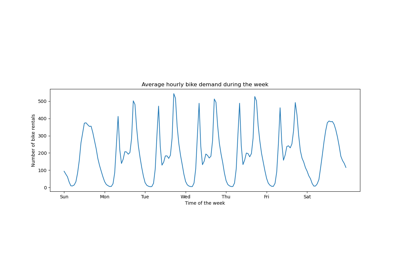Time-related feature engineering

Time-related feature engineering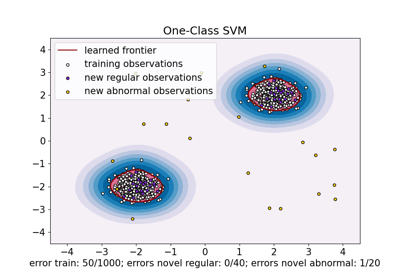One-Class SVM versus One-Class SVM using Stochastic Gradient Descent

One-Class SVM versus One-Class SVM using Stochastic Gradient Descent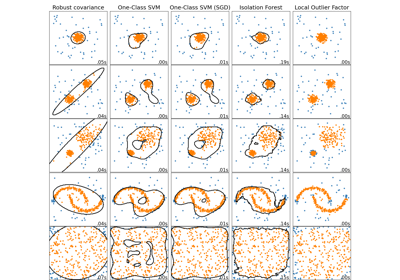Comparing anomaly detection algorithms for outlier detection on toy datasets

Comparing anomaly detection algorithms for outlier detection on toy datasets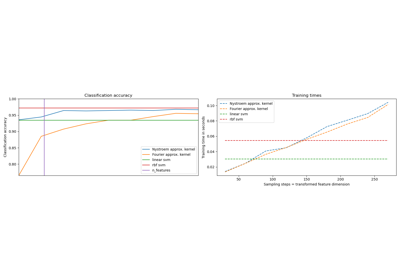Explicit feature map approximation for RBF kernels

Explicit feature map approximation for RBF kernels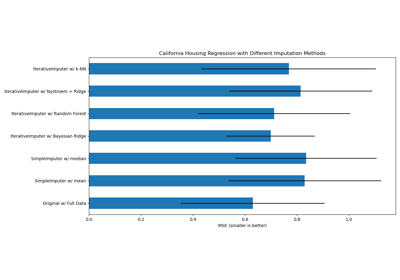Imputing missing values with variants of IterativeImputer

Imputing missing values with variants of IterativeImputer